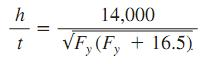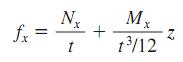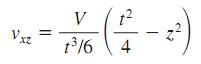Awarded as the best online publication by CIDC

# Building

Plate Girder In Buildings

For greatest resistance to bending, as much of a plate girder cross section as practicable should be concentrated in the flanges, at the greatest distance from the neutral axis. This might require, however, a web so thin that the girder would fail by web buckling before it reached its bending capacity.

To preclude this, the AISC specification limits h/t.

For an unstiffened web, this ratio should not exceed.where Fy = yield strength of compression flange,ksi (MPa).

Larger values of h/t may be used, however, if the web is stiffened at appropriate intervals.

Bearing Of Milled Surfaces

In building construction, allowable bearing stress for milled surfaces, including bearing stiffeners, and pins in reamed, drilled, or bored holes, is Fp= 0.90Fy, where Fy is the yield strength of the steel, ksi (MPa)

For expansion rollers and rockers, the allowable bearing stress, kip/linear in (kN/mm), is

Column Base Plates

The area A1, in2(mm2), required for a base plate under a column supported by concrete should be taken as the larger of the values calculated from the equation cited earlier, with R taken as the total column load, kip (kN), or

A1=R /0.70fc

Unless the projections of the plate beyond the column are small, the plate may be designed as a cantilever assumed to be fixed at the edges of a rectangle with sides equal to 0.80b and 0.95d, where b is the column flange width, in (mm), and d is the column depth, in (mm).

To minimize material requirements, the plate projections should be nearly equal. For this purpose, the plate length N, in (mm) (in the direction of d), may be taken as
N=(A1)½+0.5(0.95d–0.8b)

Bearing Plates

To resist a beam reaction, the minimum bearing length N in the direction of the beam span for a bearing plate is deter- mined by equations for prevention of local web yielding and web crippling. A larger N is generally desirable but is limited by the available wall thickness.

When the plate covers the full area of a concrete support, the area, in 2 (mm 2 ), required by the bearing plate is

A 1= R/0.35fc
Where
R=beam reaction, kip (kN),
f c= specified compressive strength of the concrete, ksi (MPa).

Stresses In Thin Shells

Results of membrane and bending theories are expressed in terms of unit forces and unit moments, acting per unit of length over the thickness of the shell. To compute the unit stresses from these forces and moments, usual practice is to assume normal forces and shears to be uniformly distributed over the shell thickness and bending stresses to be linearly distributed. Then, normal stresses can be computed from equations of the form:where
z=distance from middle surface
t=shell thickness

Mx= unit bending moment about an axis parallel to direction of unit normal force Nx

Similarly, shearing stresses produced by central shears T and twisting moments D may be calculated from equations of the form:Normal shearing stresses may be computed on the assumption of a parabolic stress distribution over the shell thickness:where V=unit shear force normal to middle surface.